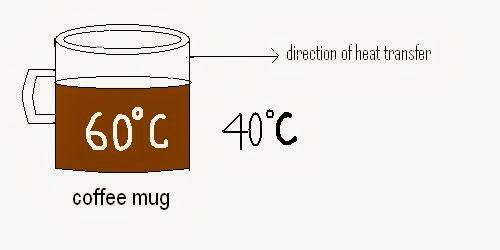# Basic Introduction About Heat Transfer

We all know that heat is the form of energy and it can be transfer from one body to another by virtue of temperature difference. The branch of science in which we study about transfer of heat is known as heat transfer.

Why should we know about heat transfer?
Heat transfer is required to know because:
• It tells us about the heat transfer rate, so we can calculate the time taken by a system to change in specified temperature.

Example:- By knowing abut the heat transfer, we can calculate in how
much time a cup of tea which is initially at 600C  takes to cool to 200C.

• Heat transfer widely used in automobile for cooling of IC engine.
• It is widely used in production of electronic equipment such as computer, DVD player, TV etc.
• It is used in various power plant such as boiler, condenser, steam developer, refrigerators etc.
How heat is transfer from a system to another system?
There are three state of material solid, liquid and gases. Heat transfer depends on the state of medium through which it is transfered. So there are three modes of heat transfer.
1. Conduction (it take place one body to another when medium molecules are in contact with body and resist to flow.)
2. Convection (it take place one body to another when medium molecules are contact with body and do not resist to flow.)
3. Radiation (it take body one body to another when bodies separated by vacuum or absence in medium.)

### Conduction:

1. Conduction is a type of heat transfer mode in which the heat transfer takes place by the flow of electron and vibration in the lattice molecules.
• Example:- Heat transfer by the lower surface of a pan to the upper surface which is placed on a burner:

2. Conduction may occur in solid, liquid and gases. In solid it is due to the flow of electron and lattice molecule’s vibration and in liquid and gases by the collision of two molecules and transfer of energy and momentum from one molecule to another.
3. Conduction is higher in solid and lower in liquid and gases.
4. The relation between the heat transfer rate with temperature difference and surface area is establish by the Fourier known as Fourier law of conduction.

#### Fourier law:

It states that rate of heat transfer is proportional to the temperature gradient exist and the surface area of the material.
q = -KA(dX/dt)
Where,
K = thermal conductivity,
q = heat transfer rate,
negative sign is used to fulfill the condition of second law of thermodynamic.
5. Thermal conductivity is the property of material which is depends upon its molecules arrangement.

### Convection:

1. Convection is the combined effect of heat transfer by conduction as well as fluid flow but it is highly depend upon the fluid flow rate so it is define as a separated mode of heat transfer.
• Example: cooling of a coffee mug placed at room temperature.2. It is the heat transfer between a solid surface and adjacent layers of liquid or gases which are in motion.

3. Convection is highly depends upon the velocity of fluid, so it is subdivided into two parts.
• Forced convection (when fluid is force towards the solid surface.)
• Free convection (when fluid is free to move or fluid is flow by virtue of density difference.)

4. The relation between convection heat transfer and the surface area of the body and temperature difference is given by the Newton known as Newton law of cooling.

#### Newton’s law of cooling:

It states that the rate of heat transfer is proportional to the surface area of the body and the temperature difference exist.

q = hA(T2 –
T1)

Where,

h = known as convection heat transfer coefficient,
A = surface area and Q is heat transfer rate.

1. Radiation is defined as the heat transfer from one body to another when the body is separated by vacuum. It is occur by emission of electromagnetic wave.
• Example:- Heat transfer from sun to earth.

2. Radiation occurs by electromagnetic waves which propagates at speed of light. It is the fastest medium of heat transfer among all.
3. The relation between heat transfer rate, surface area and temperature difference is given by the Stefan Boltzmann known as Stefan Boltzmann law.

#### Stefan Boltzmann law:

It states that a body will emit energy continuously until its temperature reaches to the temperature of surrounding. This energy is proportional to the fourth power of temperature and area of body.

q = σAT4 (for blackbody)
q = εσAT4 (for non blackbody)
Where,
ε = emissivity,
q = heat transfer rate,
σ = Boltzmann constant = 5.669*10-8 W/m2K4